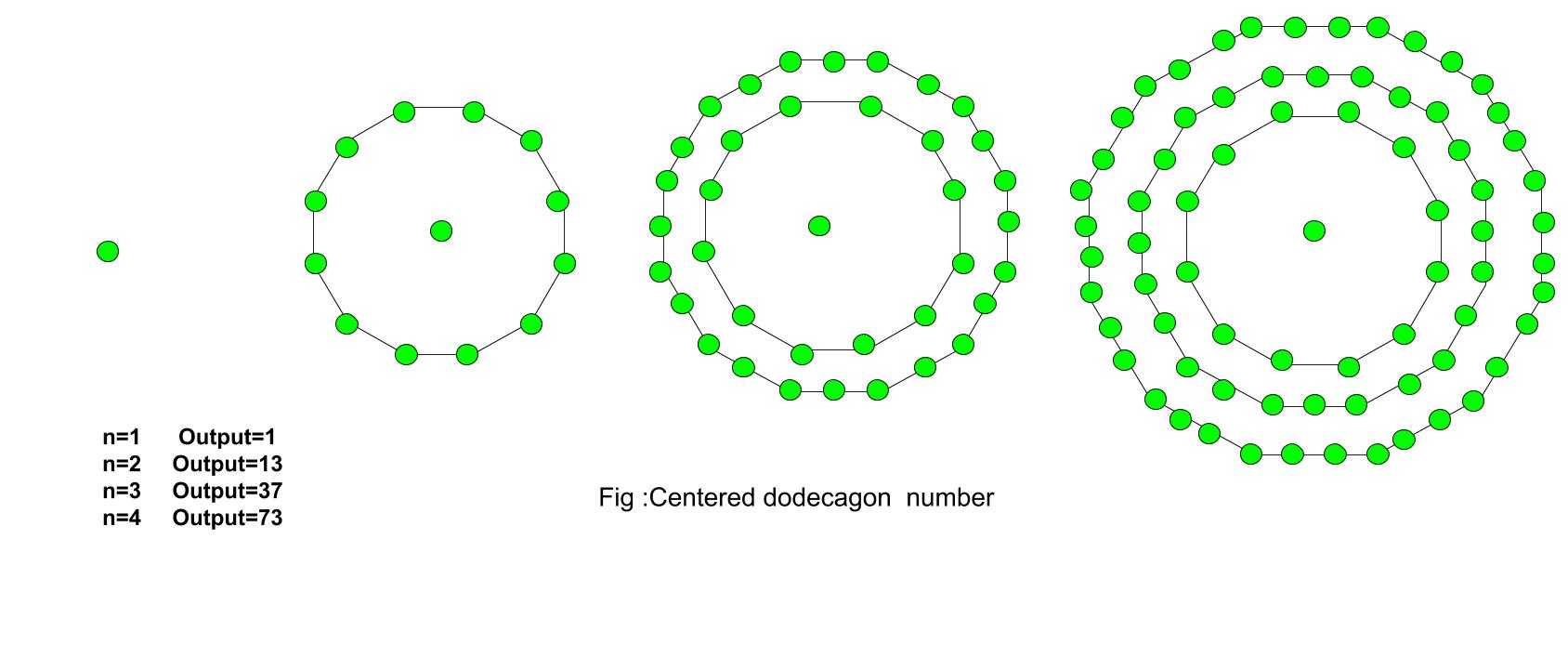Related Articles

# Centered Dodecagonal Number

• Last Updated : 13 Jul, 2021

Given a number n, find the nth Centered Dodecagonal Number.
The Centered Dodecagonal Number represents a dot in the center and other dots surrounding it in successive dodecagonal(12 sided polygon) layers.
Examples :

```Input :  3
Output : 37

Input : 7
Output :253 ```The first few centered dodecagonal numbers are:
1, 13, 37, 73, 121, 181, 253, 337, 433, 541, 661…………………..
The formula for the nth Centered dodecagonal number:

``

## C++

 `// C++ Program to find``// nth centered``// Dodecagonal number``#include ``using` `namespace` `std;` `// Function to calculate Centered``// Dodecagonal number``int` `centeredDodecagonal(``long` `int` `n)``{``    ``// Formula to calculate nth``    ``// centered Dodecagonal number``    ``return` `6 * n * (n - 1) + 1;``}` `// Driver Code``int` `main()``{``    ``long` `int` `n = 2;``    ``cout << centeredDodecagonal(n);``    ``cout << endl;``    ``n = 9;``    ``cout << centeredDodecagonal(n);` `    ``return` `0;``}`

## Java

 `// Java Program to find nth``// centered dodecagonal number``import` `java.io.*;` `class` `GFG{``    ` `// Function to calculate``// centered dodecagonal number``static` `long` `centeredDodecagonal(``long` `n)``{``    ` `    ``// Formula to calculate nth``    ``// centered dodecagonal number``    ``return` `6` `* n * (n - ``1``) + ``1``;``}` `// Driver Code``public` `static` `void` `main(String[] args)``{``    ``long` `n = ``2``;``    ``System.out.println(centeredDodecagonal(n));` `    ``n = ``9``;``    ``System.out.println(centeredDodecagonal(n));``}``}` `// This code is contributed by anuj_67`

## Python3

 `# Python3 program to find nth``# centered dodecagonal number` `# Function to calculate``# centered dodecagonal number``def` `centeredDodecagonal(n) :``    ` `    ``# Formula to calculate nth``    ``# centered dodecagonal number``    ``return` `6` `*` `n ``*` `(n ``-` `1``) ``+` `1``;` `# Driver code``n ``=` `2``print``(centeredDodecagonal(n));` `n ``=` `9``print``(centeredDodecagonal(n));` `# This code is contributed by grand_master`

## C#

 `// C# Program to find nth``// centered dodecagonal number``using` `System;``class` `GFG{` `// Function to calculate``// centered dodecagonal number``static` `long` `centeredDodecagonal(``long` `n)``{``    ` `    ``// Formula to calculate nth``    ``// centered dodecagonal number``    ``return` `6 * n * (n - 1) + 1;``}` `// Driver Code``public` `static` `void` `Main(String[] args)``{``    ``long` `n = 2;``    ``Console.WriteLine(centeredDodecagonal(n));``    ` `    ``n = 9;``    ``Console.WriteLine(centeredDodecagonal(n));``}``}` `// This code is contributed by shivanisinghss2110`

## Javascript

 ``
Output :
```13
433```

Time Complexity: O(1)
Auxiliary Space: O(1)

References
http://oeis.org/A003154

Attention reader! Don’t stop learning now. Get hold of all the important DSA concepts with the DSA Self Paced Course at a student-friendly price and become industry ready.  To complete your preparation from learning a language to DS Algo and many more,  please refer Complete Interview Preparation Course.

In case you wish to attend live classes with experts, please refer DSA Live Classes for Working Professionals and Competitive Programming Live for Students.

My Personal Notes arrow_drop_up